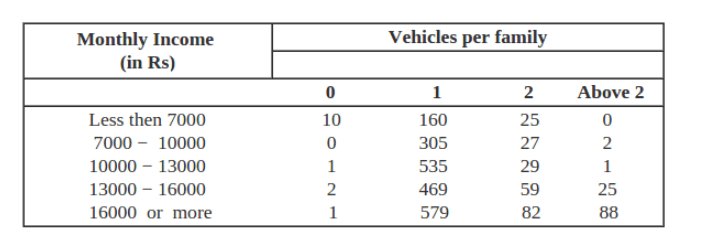# An organization selected 2400 families at random and surveyed them to determine a relationship between income level and the number of vehicles in a family.

Question.

An organization selected 2400 families at random and surveyed them to determine a relationship between income level and the number of vehicles in a family. The information gathered is listed in the table below:Suppose a family is chosen, find the probability that the family chosen is

(i) earning Rs 10000 − 13000 per month and owning exactly 2 vehicles.

(ii) earning Rs 16000 or more per month and owning exactly 1 vehicle.

(iii) earning less than Rs 7000 per month and does not own any vehicle.

(iv) earning Rs 13000 − 16000 per month and owning more than 2 vehicles.

(v) owning not more than 1 vehicle.

Solution:

Number of total families surveyed = 10 + 160 + 25 + 0 + 0 + 305 + 27 + 2 + 1 + 535 + 29 + 1 + 2 + 469 + 59 + 25 + 1 + 579 + 82 + 88 = 2400

(i) Number of families earning Rs 10000 ­− 13000 per month and owning exactly 2 vehicles = 29

Hence, required probability, $\mathrm{P}=\frac{29}{2400}$

(ii) Number of families earning Rs 16000 or more per month and owning exactly 1 vehicle $=579$

Hence, required probability, $P=\frac{579}{2400}$

(iii) Number of families earning less than Rs 7000 per month and does not own any vehicle = 10

Hence, required probability, $\mathrm{P}=\frac{10}{2400}=\frac{1}{240}$

(iv) Number of families earning Rs 13000 ­− 16000 per month and owning more than 2 vehicles = 25

Hence, required probability, $\mathrm{P}=\frac{25}{2400}=\frac{1}{96}$

(v) Number of families owning not more than 1 vehicle $=10+160+0+305+1+535+2+469+1+579=2062$

Hence, required probability, $\mathrm{P}=\frac{2062}{2400}=\frac{1031}{1200}$